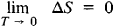# Nernst Heat Theorem

Also found in: Dictionary, Thesaurus, Wikipedia.

## Nernst heat theorem

[′nernst ′hēt ‚thir·əm]
(thermodynamics)
The theorem expressing that the rate of change of free energy of a homogeneous system with temperature, and also the rate of change of enthalpy with temperature, approaches zero as the temperature approaches absolute zero.
McGraw-Hill Dictionary of Scientific & Technical Terms, 6E, Copyright © 2003 by The McGraw-Hill Companies, Inc.
The following article is from The Great Soviet Encyclopedia (1979). It might be outdated or ideologically biased.

## Nernst Heat Theorem

a theorem of thermodynamics formulated by W. Nernst in 1906. According to the theorem, the entropy change (ΔS) approaches zero for any reversible isothermal process that occurs between two equilibrium states as the temperature T approaches absolute zero:An equivalent formulation of the Nernst heat theorem is that it is impossible to attain a temperature equal to absolute zero with the aid of a finite sequence of thermodynamic processes.

The Nernst heat theorem leads to a number of important thermodynamic corollaries; for this reason, it is often called the third law of thermodynamics.